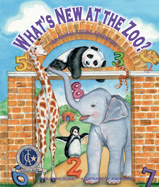Alignment to Standards for NE2,3,4 MA 2-4) 4.1.3c Solve and check a mathematical problem by using the related facts.
2,3,4 MA 2-4) 4.2.1 students will estimate, add, subtract, multiply, and divide whole numbers without and with calculators and solve word problems.
2,3,4 MA 2-4) 4.2.1a the basic facts of addition (1-20), subtraction (1-20), multiplication (1-144), and division (1-144).
2,3,4 MA 2-4) 4.6.1 students will use and interpret variables and mathematical symbols to write and solve one-step equations.
5-Mar SC5.3.1 Students will investigate and compare the characteristics of living things.
5-Mar SC5.3.1.b Identify how parts of plants and animals function to meet basic needs (e.g., leg of an insect helps an insect move, root of a plant helps the plant obtain water)
5-Mar SC5.3.2 Students will identify variations of inherited characteristics and life cycles.
K-1 MA K-1)1.1.1. the sequential order of the number system.
K-1 MA K-1)1.1.2 students will demonstrate ways of representing numbers and compare relations among numbers.
K-1 MA K-1)1.1.2a Count objects to demonstrate one-to-one correspondence.
K-1 MA K-1)1.1.2b Use comparison vocabulary (bigger, smaller, more, less, equal, higher, and lower).
K-1 MA K-1)1.1.2d Connect number words and numerals to the quantities they represent.
K-1 MA K-1)1.1.3a Identify how numbers are used in counting situations
K-1 MA K-1)1.2.1 students will demonstrate the concepts of addition and subtraction up to 10.
K-1 MA K-1)1.2.1b Recognize the symbols + and - as representing the operations of addition and subtraction.
K-1 MA K-1)1.2.1c Recognize the symbol = represents equal quantities.
K-1 MA K-1)1.2.1f Compute efficiently and accurately basic number facts for addition and subtraction.
K-2 SC2.1.1.c Select and use simple tools appropriately
K-2 SC2.3.1.c Identify external parts of plants and animals
K-2 SC2.3.2.a Describe how offspring resemble their parents

Back to Standards Page